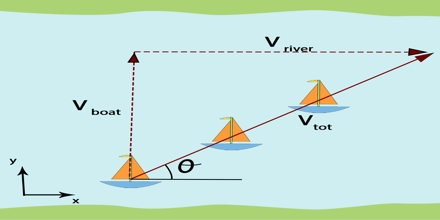# Relative Motion

Relative Motion

In order to know the position of a body another body is needed. Only then the position of the first body can be fixed with respect to the second body; otherwise it is of no use to say about the position of a body or your idea about the motion of a body does not become clear. Suppose you are sitting on a boat with a wheel to catch fish where there is no current. You see that two boats are moving fast in the same direction. After sometime you see that the two boats are moving against each other. Now you start thinking what is the velocity between the two boats moving in the same direction and between the boats moving in the opposite direction? For determining this type of motion you need another body with respect to which measurement is to be done. This second body is called the reference body or reference frame.Relative Motion is the laws of physics which apply when you are at rest on the earth also apply when you are in any reference frame which is moving at a constant velocity with respect to the earth. For example, you can toss and catch a ball in a moving bus if the motion is in a straight line at constant speed.

Generally, by the velocity of a body we understand its velocity with respect to earth. Again we can also find the velocity of a body. A with respect to another body B which is in motion on earth. It a called the relative velocity of the body ‘A’ with respect to the body B. In this case, relative velocity of ‘A’ body with reference to another body is equal to the vector difference between the two velocities, i.e., relative velocity of a body with reference to another body in motion on the earth’s surface is equal to the vector difference of the two velocities.Suppose the velocities of two bodies A and B are respectively vA and vB, then relative velocity of A with respect to B is

vAB = vA – vB

Again, relative velocity of B with respect to A is,

vBA = vB – vA = – (vA – vB)

So, vBA = – vAB